# Concept: Mensuration

RIGHT PRISM

A solid in which the top and bottom surfaces are identical polygons and the lateral (vertical) surfaces are rectangular in shape and perpendicular to the top and bottom surfaces is known as a right prism. In a right prism, the corresponding sides of the top and bottom polygons are parallel.

CUBOID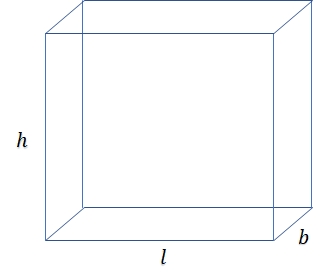• Volume (V) = lbh

• Total Surface Area (TSA) = 2(lb + bh + lh)

• Lateral Surface Area (LSA) = 2(l + b)h

• Surface Diagonals (12) = $\sqrt{{l}^{2}+{b}^{2}}$ or $\sqrt{{h}^{2}+{b}^{2}}$ or $\sqrt{{l}^{2}+{h}^{2}}$

• Body Diagonal (4) = $\sqrt{{l}^{2}+{b}^{2}+{h}^{2}}$

CUBE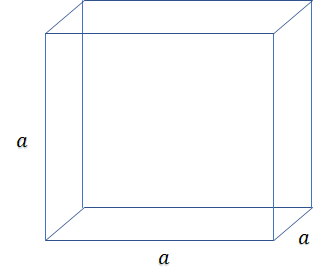• Volume (V) = a3

• Total Surface Area (TSA) = 6a2

• Lateral Surface Area (LSA) = 4a2

• Surface Diagonal (12) = a√2

• Body Diagonal (4) = a√3

CYLINDER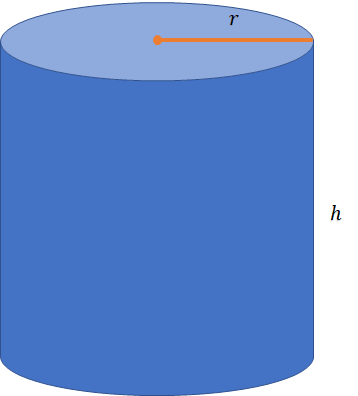• Volume (V) = πr2h

• Lateral Surface Area (LSA) = 2πrh

• Total Surface Area (TSA) = LSA + 2πr2

RIGHT PYRAMID

A right pyramid is a solid in which: The bottom surface (i.e. base) is a polygon, each vertex of which is joined with the help of slanted edges to a single vertex at the top. The lateral (slanted−vertical) surfaces are triangular in shape. The line joining the top vertex to the centre of the polygon which forms the bottom surface (also known as the height) is perpendicular to the bottom surface.

• Volume (V) = ⅓ × base area × height

• Lateral Surface Area (LSA) = ½ × base perimeter × slant height

• Total Surface Area (TSA) = LSA + base area

CONE• Volume (V) = ⅓ × πr2h

• Lateral Surface Area (LSA) = πrl

• Total Surface Area (TSA) = LSA + πr2

• Slant height (l) = $\sqrt{{r}^{2}+{h}^{2}}$

When a cone is made out of a sector of a circle of radius R• Slant height of the cone (l) = R

• Radius of the cone (r) = $\frac{𝜃}{360°}$ × R

FRUSTUM OF A CONE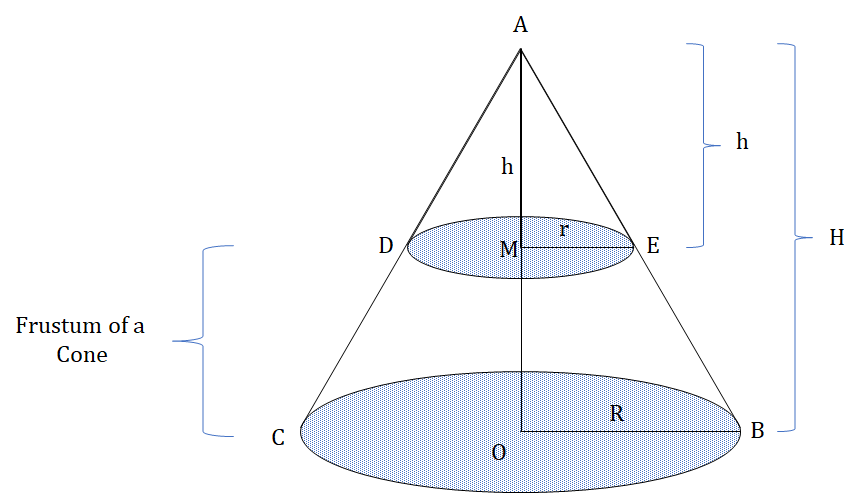• Volume (V) = ⅓ × πHf(R2 + Rr + r2)

• Lateral Surface Area = π(R + r)lf

• Total Surface Area = LSA + π(R2 + r2)

SPHERE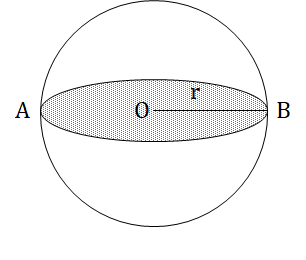• Volume (V) = $\frac{4}{3}$ × πr3

• Total Surface Area (TSA) = 4πr2

HEMISPHERE• Volume (V) = $\frac{2}{3}$ × πr3

• Curved Surface Area (CSA) = 2πr2

• Total Surface Area (TSA) = 3πr2

## Feedback

Help us build a Free and Comprehensive CAT/MBA Preparation portal by providing us your valuable feedback about Apti4All and how it can be improved.